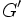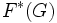# Notation for subgroup-defining functions

This is a notation page: it lists some important notation

For convenience of notation,$G$ here denotes the group on which we are computing the subgroup-defining function.

## Subgroup-defining functions

Name of subgroup-defining function Notation for single iteration Notation for$n$ iterations Associated quotient-defining function Notation for single iteration Notation for multiple iterations
Center$Z(G)$ -- (idempotent) Inner automorphism group$Inn(G), G/Z(G)$  ?
Commutator subgroup, derived subgroup$G'$,$[G,G]$$G^{''''\ldots} n$ times, or$G^{(n)}$ Abelianization$G^{ab}$ -- (idempotent)
Fitting subgroup$F(G)$ -- (idempotent) Fitting quotient$G/F(G)$ -- (idempotent)
Frattini subgroup$\Phi(G)$$\Phi^n(G)$ Frattini quotient$G/\Phi(G)$ -- (idempotent)
•$Soc(G)$ denotes the socle of$G$
•$W(G)$ denotes the Wielandt subgroup of$G$
•$O(G)$ denotes the Brauer core of$G$
•$E(G)$ denotes the layer of$G$
•$F^*(G)$ denotes the generalized Fitting subgroup of$G$
•$L(G)$ denotes the 2-layer of$G$